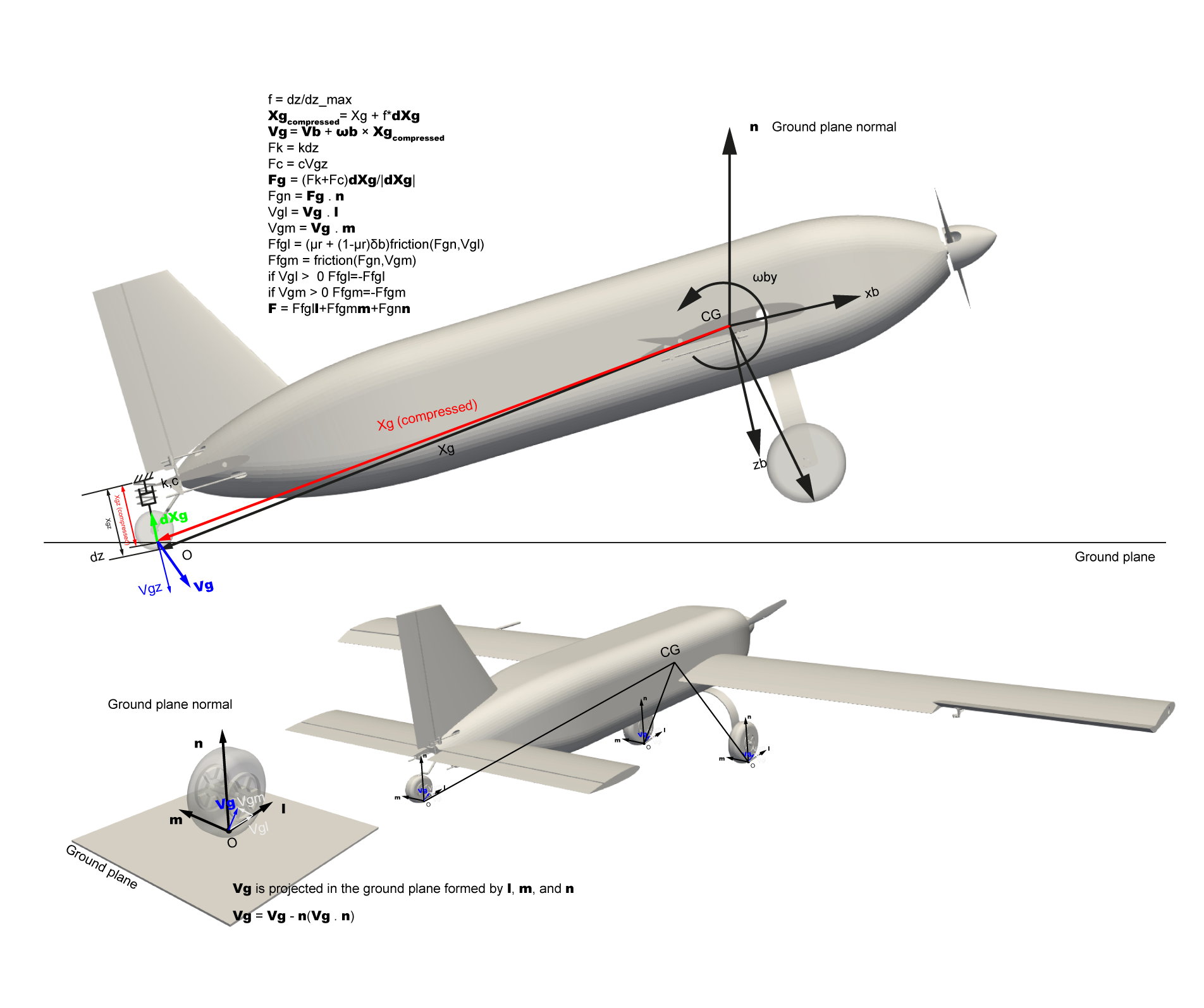# Aircraft ground interaction model

An aircraft ground interaction model used in a flight simulation is presented below. Each of the aircraft's landing gears is represented by a spring and a damper. The stiffness coefficient of the spring is denoted by k, and the damping coefficient of the damper is denoted by c. The landing gear is in contact with the ground plane if its uncompressed z-location (in body coordinates) is below the ground plane. If the z-location of the landing gear is below the ground plane, the ground plane contact point has to be determined. The ground plane contact point is obtained by determining how much the landing gear needs to compress in the z-direction of the body-fixed coordinate system. To determine how much the landing gear needs to compress, the z-location of the fully compressed landing gear (Xgb+dXgb) is obtained. The Xgb vector gives the location of the landing gear, and the dXgb vector gives the compression direction and maximum compression (e.g. dXgb={0,0,-dzmax}). The dot product of Xgb+dXgb with the ground plane normal nb added to the dot product of the aircraft location vector XeNED with the ground plane normal nNED gives the z-location of the compressed landing gear relative to the ground plane (in body coordinates). The same steps are used to obtain the z-location of the landing gear without compression.

Note: Superscript b denotes the body-fixed coordinate frame and superscript NED denotes the North-East-Down coordinate frame. The ground plane normal is defined as nNED={0,0,-1} and nb=DCMbenNED, where DCMbe is the rotation matrix from the NED to the body-fixed coordinate frame. You can refer to the schematic below for more detail:If the z-location of the fully compressed landing gear is below the ground plane this means that the landing gear is fully compressed (the compression ratio, f, is equal to 1). If the z-location of the fully compressed landing gear is above the ground plane, the compression ratio is obtained from the following equation: z/(z-zcompressed) where z is the z-location of the uncompressed landing gear. The compressed landing gear location (ground plane contact point) is obtained from the following equation: Xgbcompressed=Xgb+f*dXgb. After obtaining the compressed landing gear location, the velocity at this location is obtained from the following equation: Vgb=Vbb+ωbbxXgbcompressed. The spring and damper forces are then be obtained. The gear force Fgb is obtained by multiplying the dXg vector (the vector is first normalized) by the sum of the spring and damper forces. A local right-hand coordinate system (lb, mb, nb) is constructed from the ground normal nb and the x-axis of the body-fixed coordinate system xb={1,0,0}.

• mb=nb x {1,0,0}
• lb=mb x nb
The l-and-m components (Vgl and Vgm) of the landing gear velocity Vgb are obtained by taking the respective dot products. The normal component of the gear force Fgn is obtained from the dot product of the gear force Fgb and the ground plane normal nb. The friction force components along the l-and-m axes (Ffgl and Ffgm) are calculated from the following equations:
• Ffgl = (μr+(1-μr)δb)friction(Fgn,Vgl),
• Ffgm = friction(Fgn,Vgm),
where friction(Fn,V) is a function calculating the friction. ``` Ff = friction(Fn,V) V=abs(V) if V < 0.1 Ff = 1/0.1 x V x Fn x μs else Ff = Fn x μd end ```

If the Vgl and Vgm velocity components are positive, the friction force components are multiplied by -1.0. The total gear force is obtained from Fb=Ffgllb+Ffgmmb+Fgnnb.

Values for the stiffness and damping coefficients k and c are obtained from:

• k=(1/dz_max)*m*g*10,
• c=2*sqrt(k*m),
where m is the aircraft mass, and dz_max is the maximum landing gear deflection. The number 10 is a multiplier (i.e. the gear will deflect dz_max under 10-times the aircraft weight). Both dz_max and 10 need to be adjusted depending on the aircraft type/model.

Below is a Simulink model implementing the ground interaction model using C++ S-functions and the 6DOF ECEF Equations of Motion block. The model and the mex file of the C++ S-function can be obtained from: